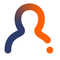cancel
Showing results for
Did you mean:
cancel
Showing results for
Did you mean:

## How to draft Arcs and Circles in ArchicadGraphisoft

### The Arc/Circle Tool

To explain the different methods of how to create an arc, we now change to the Arc/Circle Tool.

This tool includes two main Geometry Methods:

• Arc/Circle
• Ellipse.

If you click on the icon and hold the left mouse button down, you can select the appropriate Geometry Method within the two main methods:

#### Geometry Method: Arc / Circle

It creates an arc based on the center point and the radius.
1. The first click defines the center point.

2. Move the cursor and click again which will define the radius.

To define the arc length or rather the angle of the arc, move the cursor either to the right or to the left side.
3. The third click completes the arc.

Note that to create a full circle, the start point must also be the endpoint.
which means the second and the third click will be on the same point.

Circumference
It creates an arc based on three points of the arc circumference.
1. The first click defines the start point of the arc.
2. The second and the third clicks define a triangle and therefore the circumference.
3. The fourth click completes the arc by determining the arc length.

Tangential
It creates an arc based on three existing tangential edges or points. This method always creates a complete circle (360°). There are three different options to use this Geometry Method:

1 tangential edge and 2 points
First, draw three single lines as shown in the picture below.

The first click defines the tangential edge we want to use as a reference. Make sure that the cursor turns into the Mercedes shape before clicking. We'll use the endpoints of the other two lines as reference points. (The cursor turns into a black pen when finding an endpoint. You can learn about the smart cursor of Archicad in here.)

If there are multiple solutions, the eyeball-shaped cursor appears and the ghost contour of the arc flips from one position to the other as you move the mouse around. Click to complete the circle.

2 tangential edges and 1 point
First, draw three single lines as shown in the picture below.

We use both lines at the bottom as the tangential edges and the endpoint of the remaining line above as the reference point.

By using the Eyeball cursor, you can select which circle will be created.

3 tangential edges
1. First, draw a triangle out of single lines as shown in the picture below.

2. This time, you select three tangential edges as references for the arc. Click on each of them.
3. Use the eyeball-shaped cursor to define the right direction for the circle.

#### Geometry Method: Ellipse

To draw an elliptical arc or a full ellipse, choose the Arc/Circle tool in the Toolbox and one of the three Geometry Methods offered by the second icon in the Info Box:

• The Diagonal Ellipse method creates an Ellipse constrained into a rectangle.
• The Semi-Diagonal Ellipse method works the same way as the Diagonal method. In this case, you define the imaginary rectangle by its center point and the endpoint of its semi-diagonal.
• The Ellipse Radii method defines an elliptical arc by the major and another radius of an ellipse and the angle of the arc.

Steps of the Ellipse Radii method:
1. The first click defines the center point of the ellipse.
2. Choose the orientation of the major (first) radius by clicking again.
3. You get the rubber band line of the second radius, which you can stretch to different sizes and angles. Notice the accompanying ellipse that fits the length of the two radii. If you stretch the second radius too far away or right in line with the major (first) radius, you cannot draw the ellipse.
4. In the final phase, Archicad holds the ellipse while you define the side angle of the arc. First, you use a rubber band line to define the side where the angle starts from. Then you define a different side that closes the angle, while Archicad follows the angle with the Elliptical Arc. Neither side of the angle remains visible when the final arc is drawn.

Contributors
Version history
Last update:
‎2021-11-26 01:28 AM
Updated by: